# Time Series ARIMA Forecasting Using R

## Time Series Forecasting Using R: A Starter Pack

#### Some basic theoretical ideas needed before we proceed:-

Time Series Data- A time series is a set of observations on the values that a variable takes at different times. Such data may be collected at regular time intervals, such as daily (e.g., stock prices, weather reports), weekly (e.g., money supply), monthly [e.g., the unemployment rate, the Consumer Price Index (CPI)], quarterly (e.g., GDP), annually (e.g., government budgets), quinquennially, that is, every 5 years (e.g., the census of manufactures), or decennially (e.g., the census of population).

Stochastic Process- A random or stochastic process is a collection of random variables ordered in time.

Stationary Stochastic Processes- A stochastic process is said to be stationary if its mean and variance are constant over time and the value of the covariance between two time periods depends only on the distance or gap or lag between the two time periods and not the actual time point at which the covariance is computed.

Nonstationary Stochastic Processes- A stochastic process is said to be nonstationary if at least one of the characteristics of it from among its mean or variance or the value of the covariance between two time periods depends on the actual time point at which it is being computed.

Why are stationary time series so important- This is because if a time series is nonstationary, we can study its behaviour only for the time period under consideration. Each set of time series data will, therefore, be for a particular episode. As a consequence, it is not possible to generalize it to other time periods. Therefore, for the purpose of forecasting, such (nonstationary) time series may be of little practical value.

Autocorrelation Function (ACF)- One simple test of stationarity is based on the so-called autocorrelation function (ACF). The ACF at lag k is given as = (Covariance at lag k/Variance).

Augmented Dickey-Fuller Test (ADF Test)- Augmented Dickey-Fuller test (ADF) tests the null hypothesis that a time series sample suffers from Non-Stationarity vs. the alternative hypothesis of Stationarity or Trend-Stationarity.

Exponential Smoothing Methods- These are essentially methods of getting a suitable curve to historical data of a given time series. There are a variety of these methods, such as single exponential smoothing, Holt’s linear method, and Holt-Winters’ method and their variations.

Auto Regressive Model (AR Model)- The autoregressive model specifies that a time series variable depends linearly on its own previous period values and on a stochastic term. Thus the model is in the form of a stochastic difference equation. The autoregressive model is not always stationary.

Moving Average Model (MA Model)- The Moving Average Model is a common approach for modeling univariate time series. The moving-average model specifies that the output variable depends linearly on the current and various past values of a stochastic term. Contrary to the AR model, the finite MA model is always stationary.

Auto Regressive Integrated Moving Average Model (ARIMA Model)- Auto Regressive Integrated Mmoving Average (ARIMA) Model is a generalization of an ARMA Model. Both of these models are fitted to time series data either to better understand the data or to predict future points in the series (forecasting). ARIMA models are applied in some cases where data show evidence of non-stationarity, where an initial differencing step (corresponding to the “integrated” part of the model) can be applied one or more times to eliminate the non-stationarity.

#### In the next portion, we discuss the hands-on techniques required to perform a Time Series Analysis in R.

At first, we need to Call up the library named “forecast” required later to perform predictions.

The next step is setting the working directory of the data set under consideration.
The required path for my system is:-

So, we use the function “setwd( )” along with the above relevant path, to set the working directory.

The name of the required data set in my analysis is “Sales.csv”, i.e. a CSV file. If anyone needs to get access to this data set, get it from the link below link for dataset – Sales.csv.

So we create a space in R to read and store the entire data from the “Sales.csv” file. Thus we require R to read the required CSV file using the ccommand: read.csv(“sales.csv”). After reading the data, we want R to store it in a space named “data”. So, “data” is the name of the data set in R, henceforth.

Now we will be using the data set named “data” for our analysis. By default “data” has been created by R as a Data Frame. Just to verify we check for the class of “data” using the following command.

On execution of the above command, it shows “data.frame”. Next, we need to look at the contents of the data frame named “data”. Since it is not necessary at all to go through all the rows of records in “data”.
So we just want to get an idea by looking at the first few rows of “data”. So we use the function “head( )”, which returns the 1st 6 rows of records from “data”.

On execution, we see that “data” contains 2 columns/variables. The 1st one is “Month.Year” having values like “Jan-03”, “Feb-03”, etc. So it is the time variable of our data frame. The 2nd variable is “Sales”, having records like “141”, “157”, etc.

So this is the variable/series varying over time which we need to analyse. This series viz. “Sales” is a continuous variable, as evident from its data records. Next, we plan to rename the column heads of “data” as per our own convenience.

So, we choose to rename the original time variable “Month.Year” as “Date”. And the variable “Sales” is to be renamed as it is. We use the “names( )” function to rename both the columns of “data”.

So we specify as an option while renaming, that we want to start with the 1st column head. And then proceed on renaming till the 2nd column head. So, we use “names(data)[c(1:2)]” .

Again to verify whether the renaming is done successfully we check the content of “data” again.

On execution, we see the renaming has been successfully done. Now our next task is to instruct R to convert this data frame into a time series structure. So, we use the “ts( )” function with its relevant arguments on the data frame “data”. The conversion of the original data frame “data” into Time Series mode is done as follows.

We see in the above process, while using the function “ts( )”, we specify the 2nd column of “data”, i.e. “Sales” as the series under consideration and also indicate to R, that the starting point of the series should be “(2003,1)”, i.e. the 1st month of the year 2003. Also, the frequency was set to be equal to 12, indicating that all the 12 months of each year are to be considered.

Now just for the sake of knowing the class of “data” after this conversion we do the following.

We see that the class has changed to “ts”, indicating a Time Series structure. To understand the differences newly created in the Time Series structure “data”, after it has been tabulated in Time Series mode, we return the entire data frame.

On execution, we see that the earlier representation of “data” has now been changed. Now, it has a more structured and bi-variety tabulated look. Next, we go for an initial visual analysis of the series “Sales” from “data”. Actually, this will help us to get a crude idea about the nature of the series “Sales”.

So, we plot the “Sales” data points against the corresponding values of the time variable.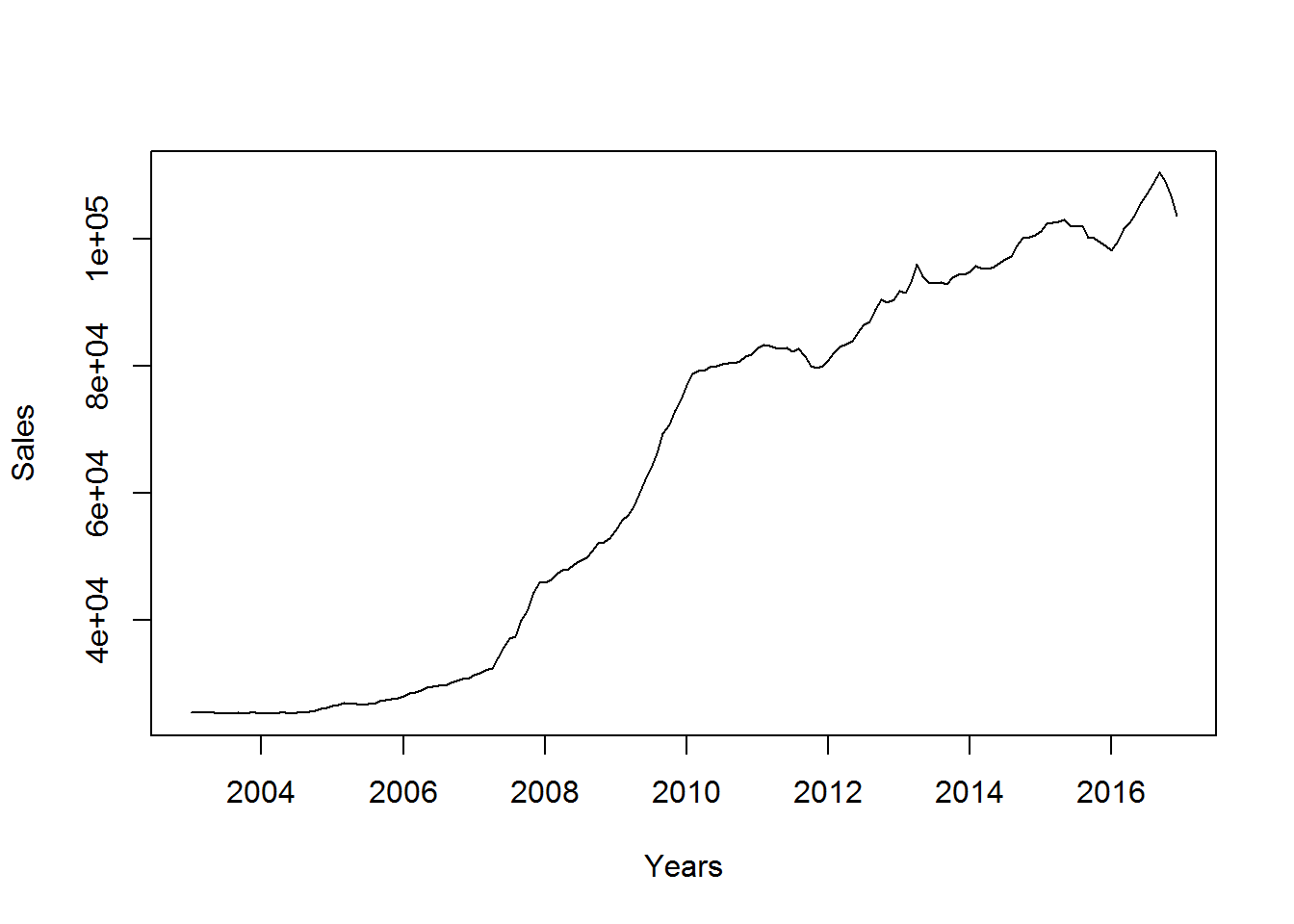Here we re-label the X-axis as “Years” and the Y-axis as “Sales”. We see from the plot that the values of the series “Sales” are gradually increasing over time. Moreover, there are some ups and downs in the path of the series “Sales” over time.

Again, these ups and downs gradually increase in magnitude over time, specifically from 2011. This is quite evident from the heights of the spikes created starting from the year 2011 and on. So, we can apparently say that this series has an upward rising trend.

Also, it shows that some kind of seasonality from the year 2011 until the very end. During some months of each year starting from 2011, the “Sales” go up drastically, as compared to its values during the other months of that year.

The magnitude of this seasonal change in “Sales” taking place each year also increases over the years.
This is evident from the fact that the heights of the spikes go on increasing from one year to the next and so on.

So, it may be apparent that the series “Sales” taken up here suffers from non-stationarity as well. But we need to conduct the stationarity test of the series “Sales”, in order to get certified. So we conduct the Augmented Dickey-Fuller (ADF) Test to check for stationarity.

For this, we specifically require to call up the R library “tseries” and then conduct the test.

Here while conducting the ADF Test on the series “Sales” of “data”, we pre-specify the alternative hypothesis of the test to be renamed as “stationary”.

So, our ADF Test validates for, Ho: The series is Non-Stationary. vs. H1: The series is stationary. On execution of this test, we see from the results that ADF has automatically considered 5 to be the appropriate order of lag for the series “Sales” at level.

However, the results of this test show that the p-value = 0.9318 (i.e. > 0.05). So at a default level of significance of 5 %, this test indicates that the observed p-value is much higher than the critical p-value here.

This indicates that here we have to reject the Alternative Hypothesis, but fail to reject the Null Hypothesis.
Thus it is clear that the series “Sales” suffers from Non-Stationarity at level. Proceeding with this Non-Stationary series for further analysis is not viable.

Had this series been found to be stationary at the level only, then we could have proceeded with it. So, now we need to go for differencing the series “Sales” and then conducting the ADF Test to check if the differenced series is Stationary or not.

If after taking the 1st difference of the series “Sales”, we still find that the ADF Test indicates this 1st differenced series to be Non-Stationary also, then we need to consider the 2nd order difference of the series “Sales” and again check for its Stationarity using ADF Test.

This process should be continued until we obtain stationarity at some higher level of difference. So, this time we go for a Time Series plot of the 1st difference of “Sales” from “data”. This will again help us in getting a visual idea.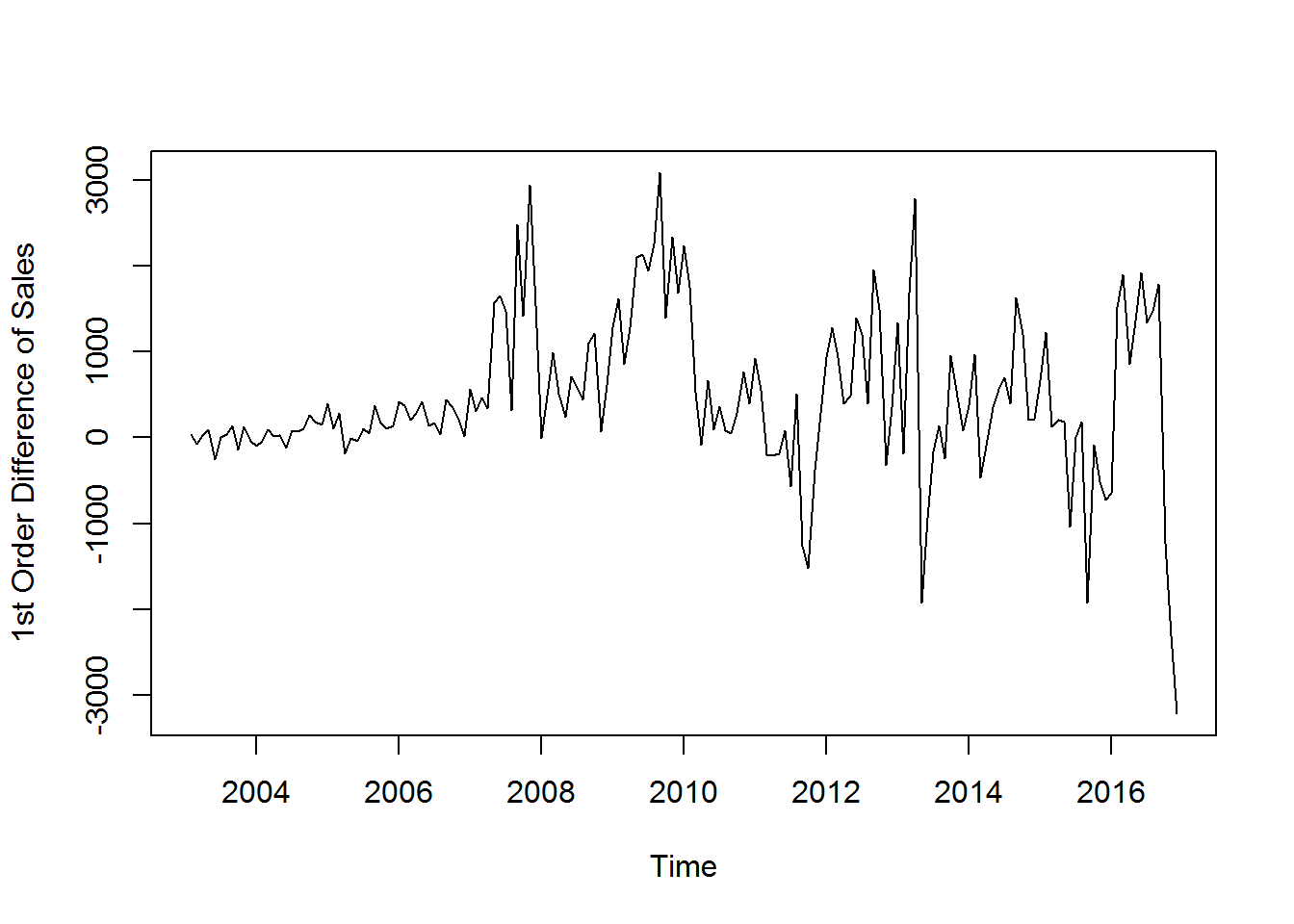Here, we use “diff(data)” to indicate the 1st order difference of “Sales” from “data”, to be considered as the variable for plotting against time. We re-label the Y-Axis as “1st Order Difference of Sales”, just for our convenience.

The graph indicates that the 1st order difference of “Sales” when plotted against time, shows no trend in the Long Run. However, it exhibits some up and down movement in its path over the years.
Thus some steep spikes are created over time.

Again, the magnitude of fluctuation represented by these spikes increases gradually over the years. This implies although the mean behaviour of this series is time-invariant, the variation shown by it over the years is certainly time dependent.

So again, it is suspected that this 1st differenced series too may be Non-Stationary. But, still, we conduct the ADF Test to get a strong verification. So, we first store the 1st order differenced series of “Sales” in a different TS structure.

We have a look at the get up of this new TS structure named as “data1”. Then we conduct the ADF Test on the differenced series of “Sales”, which has been separately stored in this new TS structure named “data1”.

On execution, we again see that the appropraite lag length has been automatically chosen as 5.
The p-value here is 0.1612 (i.e. > 0.05). This indicates that at 5 % level of significance, we need to reject the Alternative Hypothesis again.

Thus it is evident that this 1st order differenced series of “Sales” is Non-Stationary too. We cannot proceed with this series even. So, we go for 2nd order differencing of “Sales” and conduct ADF Test on it, We use the same process as above.

First, we plot the 2nd order differenced series of “Sales” and then try to get a visual idea.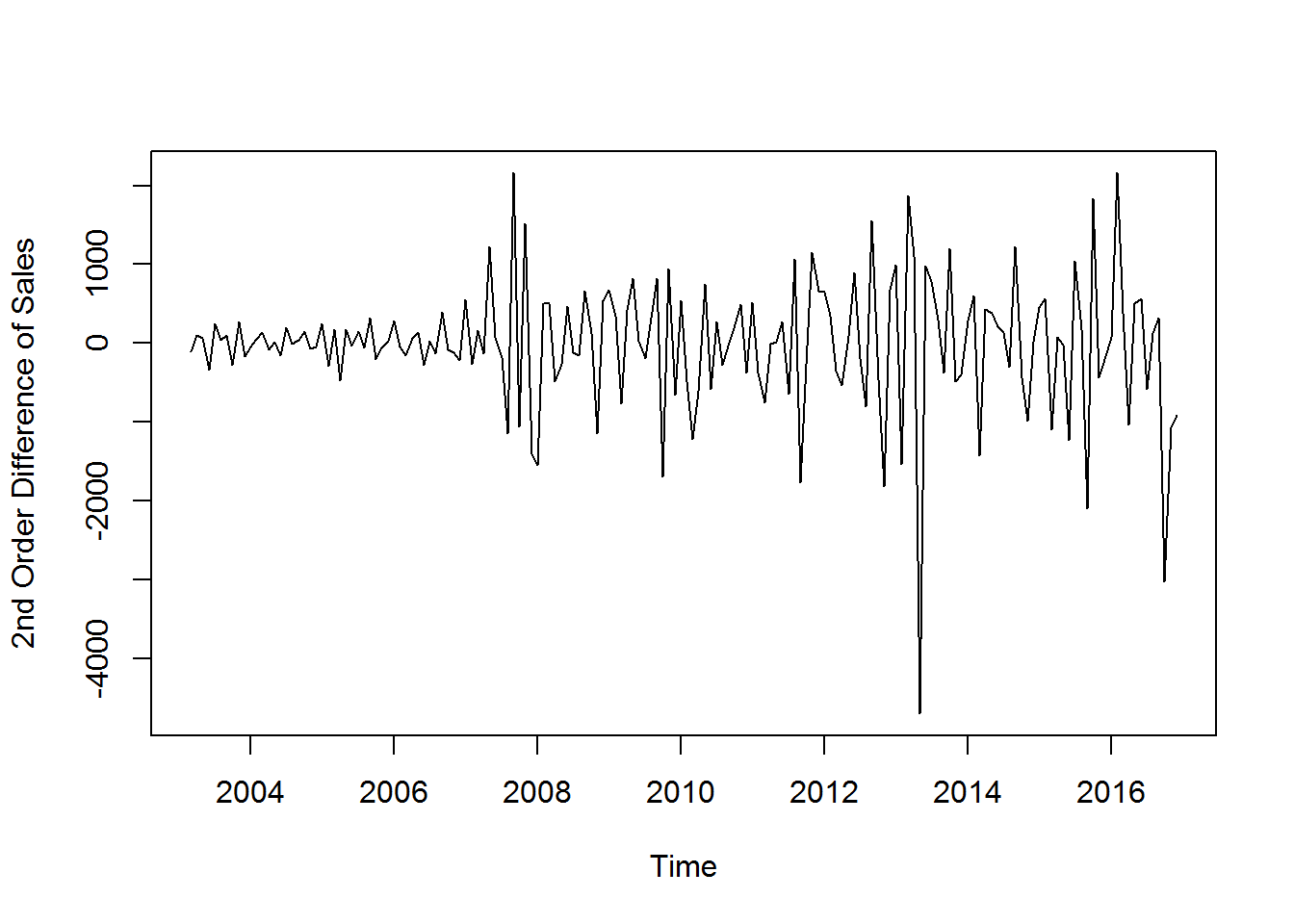On execution, from the graph, we again find that there is no presence of Long Run trend. However, the spikes/fluctuations are still there showing an increase in their magnitude over time. So, we suspect presence of time component in the variance, if not in trend. Again, there is a chance of Non-Stationarity. So we test it.

On execution, we see that the appropriate lag order is 5 again. But this time the p-value = 0.01 (i.e. < 0.05).

So, at 5 % level of significance, we need to reject the Null Hypothesis. Thus we fail to reject the Alternative Hypothesis in this case.So, the 2nd order differenced series of “Sales” is Stationary. This goes against the usual visual inference that we had made earlier from the graph.

So, this is where testing proves to be necessary for verification. Thus we can proceed further with this stationary series for further analysis. But there is another factor we need to take care of.

We have seen from all the previous graphs that there were some unevenness in the fluctuations shown by the different ordered differenced series of “Sales” as well as the series “Sales” at level. This uneven fluctuation/variance if corrected for could improve our results further. So we try out with a logarithmic transformation of the series of “Sales” at level. We plot it first and then try to carry out the further steps as before.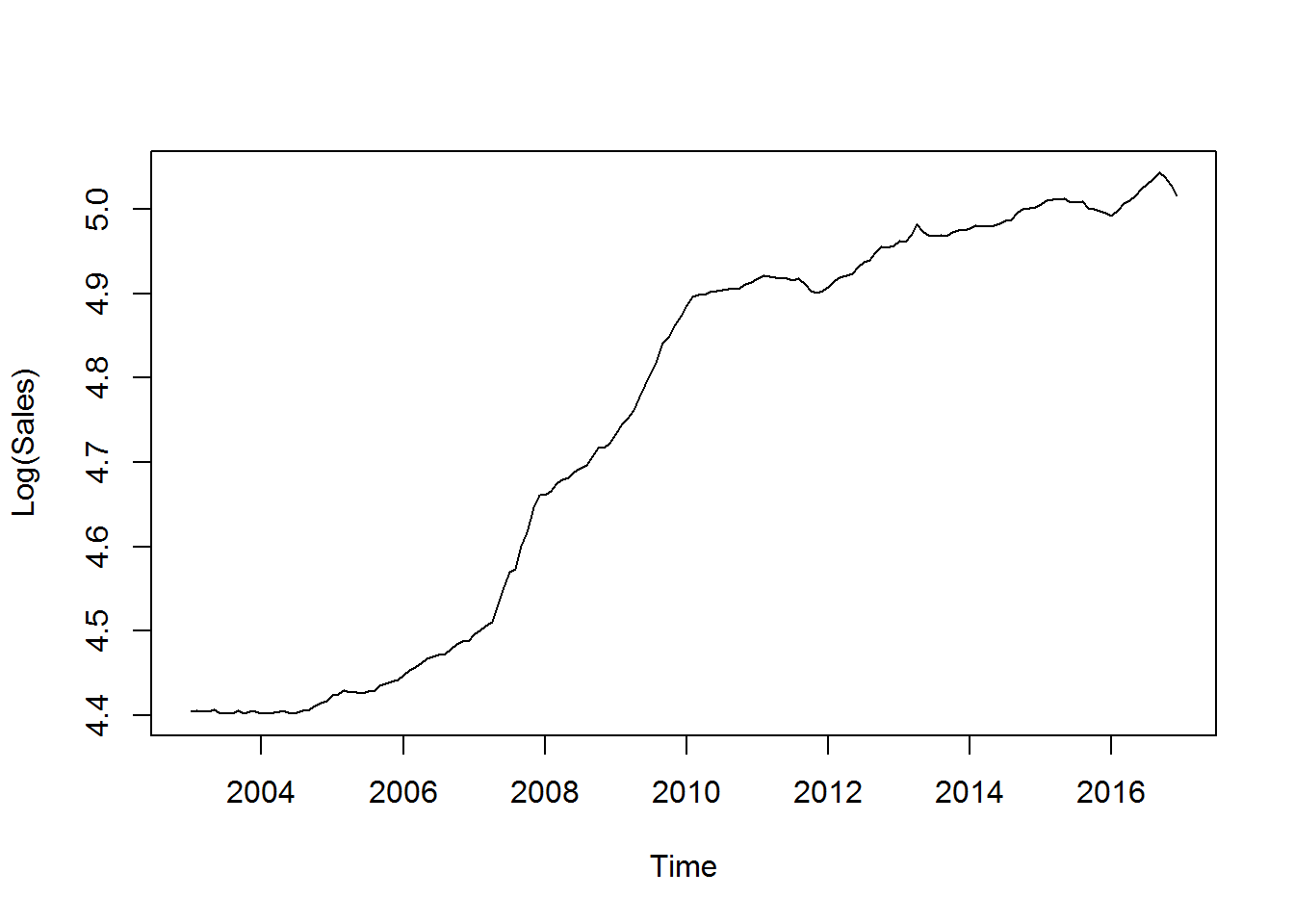On execution, we see from the graph that there is a Long term upward trend. However, the fluctuations/spikes have significantly smoothened up as compared to the graph of the orginal series “Sales” at level. Next we go for the test of stationarity.

On execution, we see from the test results that the p-value = 0.9805 (i.e. > 0.05). So at 5 % level of significance, we have to reject the Alternative Hypothesis. Thus this series is Non-Stationary and we cannot proceed with this series futher. We now take differences as before and proceed thereafter.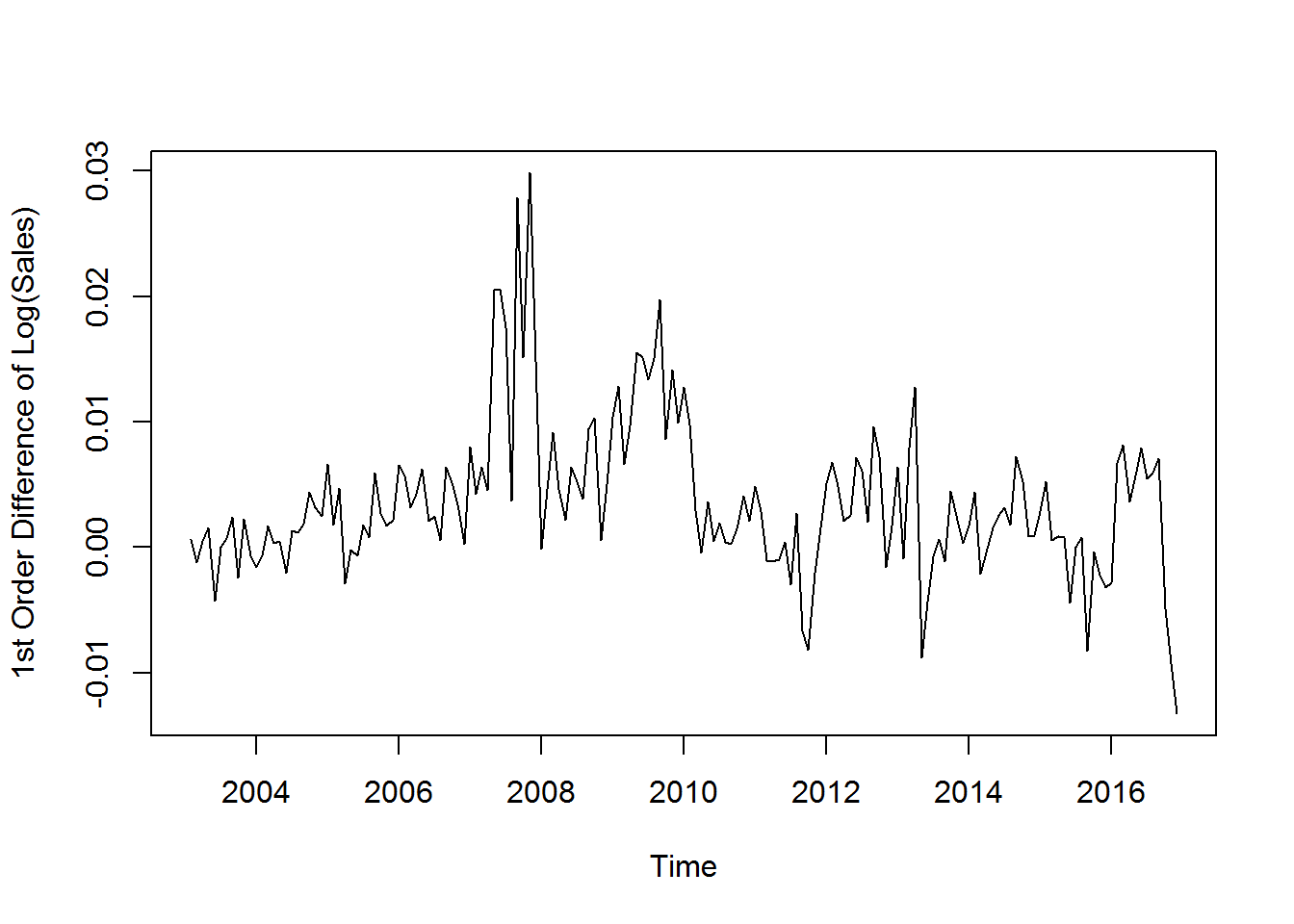The graph shows that apparently there is very little downward trend over time. The fluctuations/spikes are still there and very haphazard in nature. We conduct the ADF Test as before, to check for Non-Stationarity.

On execution, we see from the results that the p-value = 0.2463 (i.e. > 0.05). Thus at 5 % level of significance, we reject the Alternative Hypothesis. We infer that this series is again Non-Stationary.
And we cannot proceed further with this. Next, we consider the 2nd order difference of the series “Log(Sales)” and proceed further.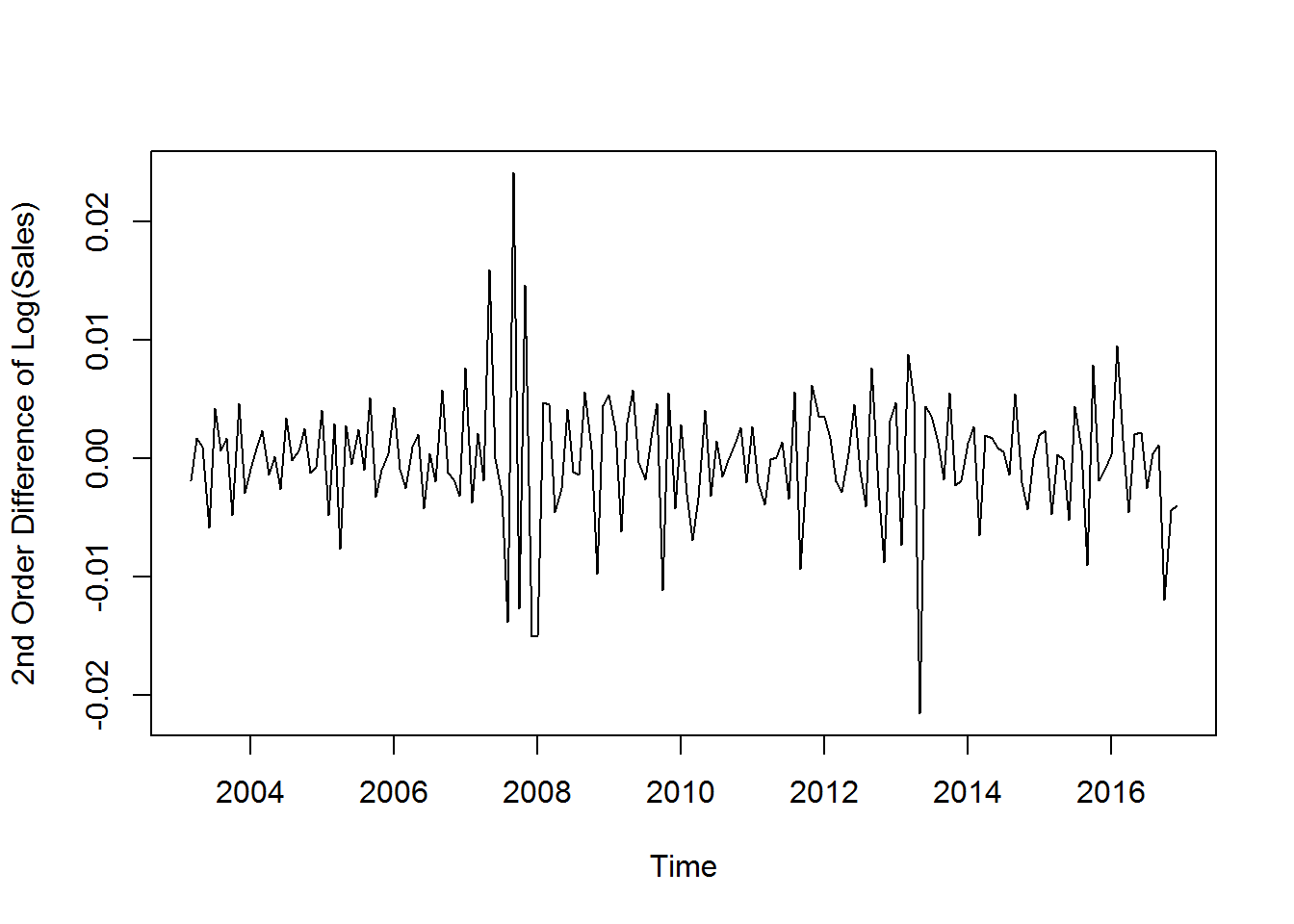From the graph, it is apparent that there is no Long Run trend. And above all the fluctuations have eased down a lot, reducing the unevenness. We check for Stationarity of this.

On execution, we see that the test results give us a p-value = 0.01 (i.e. < 0.05). So, at 5 % level of significance, we fail to reject the Alternative Hypothesis. This implies that the 2nd Order Difference of the series “Log(Sales)” is Stationary. Thus we can proceed further with this series. Till now we have finalised two different statioanry series for further analysis.

They are:- 2nd Order Difference of the series “Sales” and 2nd Order Difference of the series “Log(Sales)”
Now we will consider further analysis with these two series separately. Ultimately we will keep that one only which shows a better fit at the final stage.

Our next task is to find out the most appropraite Model for the above two series. For that we at first need to create the ACF and PACF plots for both separately.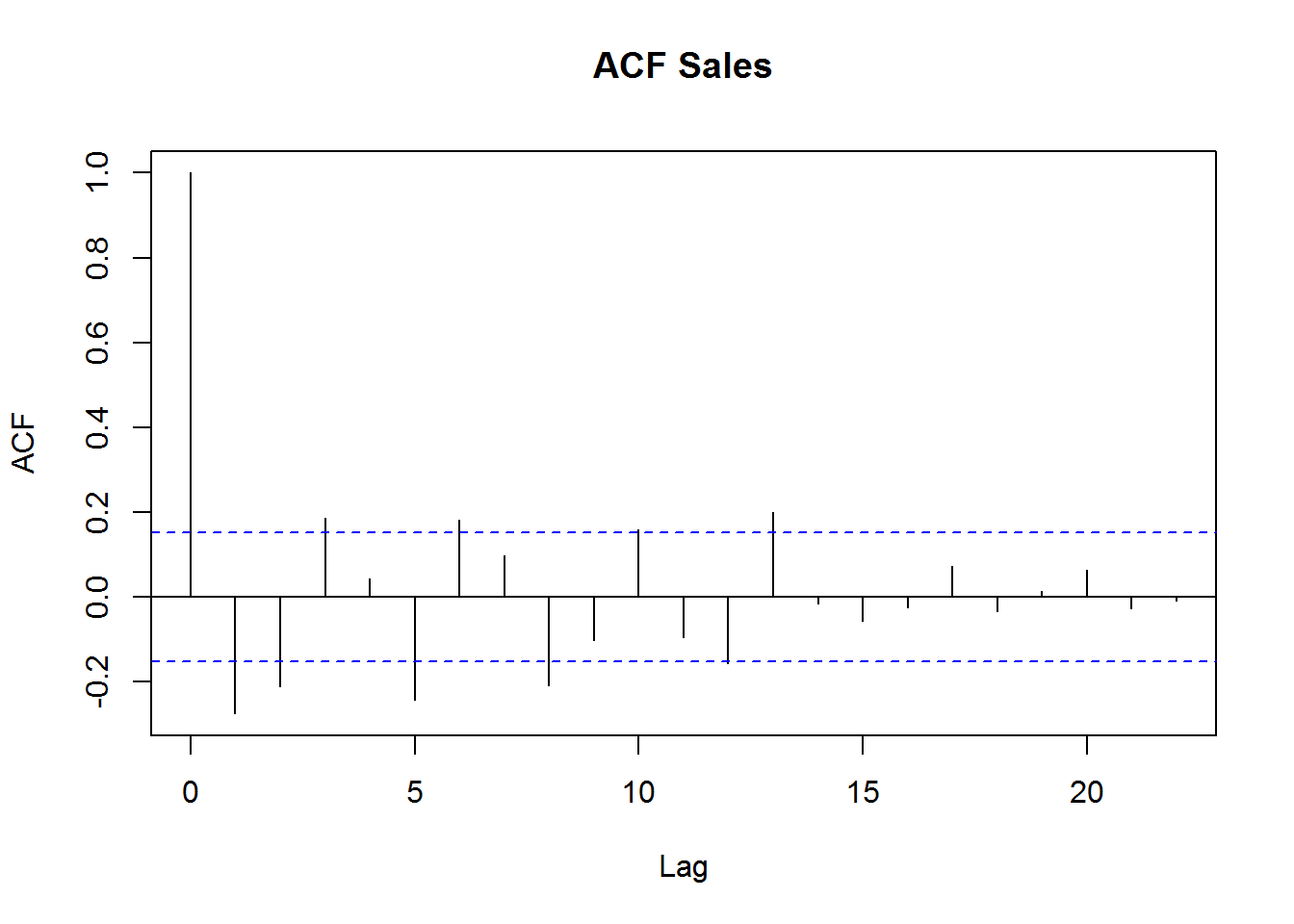We see from the ACF Plot, that the order of Moving Average is expected to be 0. This is because the last spike which is significantly outside the limits is at lag 0.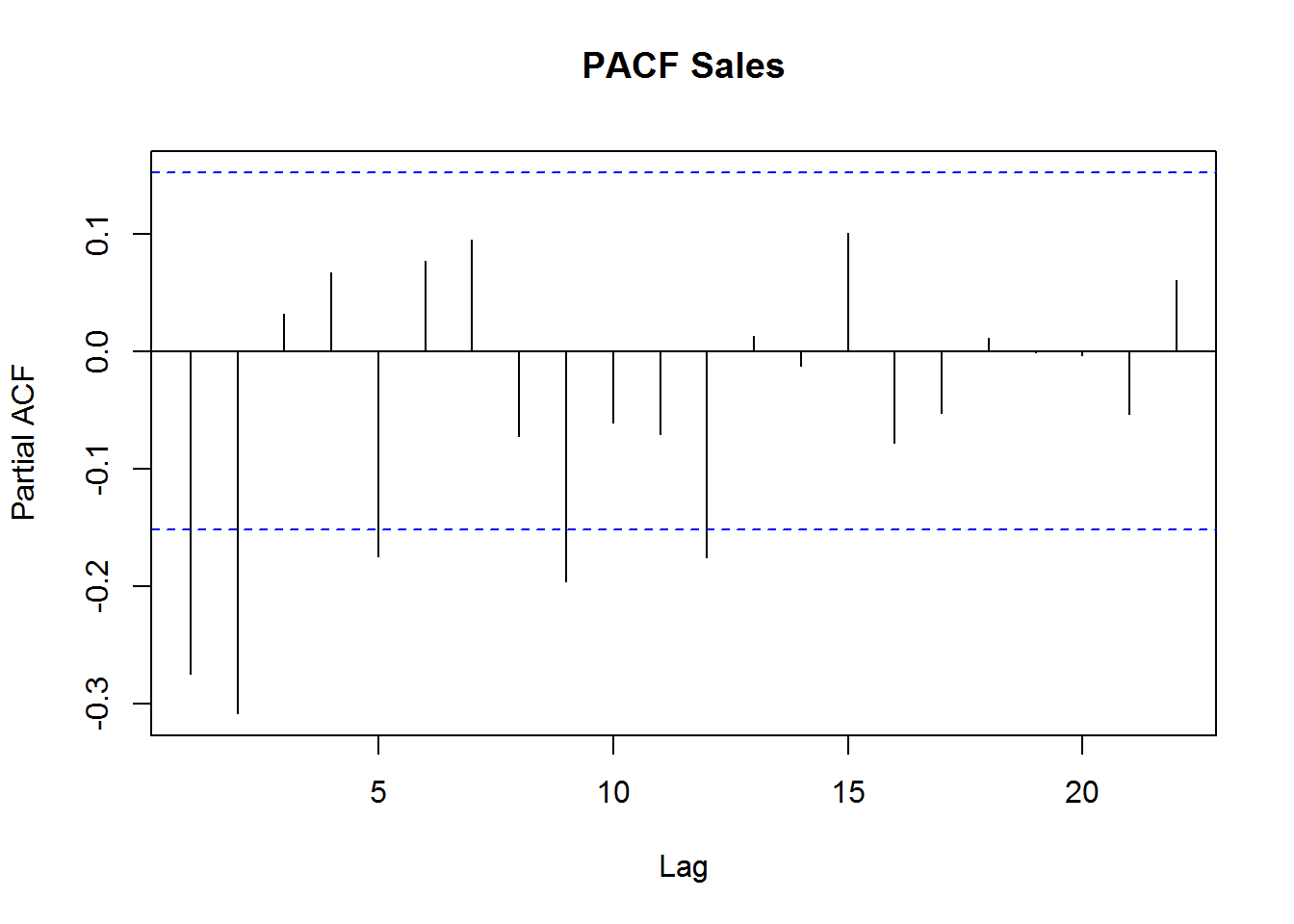We see from the PACF Plot, that the order of Auto Regression is expected to be 2. This is because the last spike which is significantly outside the limits is at lag 2. So we expect the model to be ARIMA(2,2,0)
The order of integration was previously determined as 2. We now fit the ARIMA model as per our expectations.

Here we fit the ARIMA(2,2,0) model on the 1st order difference of the series “Sales”. This is because though the order of integration is 2, i.e. we need to work with the 2nd order difference of the series “Sales”, the ARMA process requires one lag lower order of integration while operating. Thus we effectively need to model the 1st order differenced values of the series “Sales”.

## Fitting appropraite models

Next, we look at the summary of the fit stored under the name “ARIMAfit”.

From the summary results, we get the various coefficients of the model equation fitted. We just take a note of the values of the different “Training set error measures” given. These Error Measures help us to compare between two or more fitted models. The model with the lowest error values is considered to be the best fit.
However, the above ARIMA(2,2,0) model was fit on the basis of rough observations done from the ACF and PACF plots.

They may not be accurate always. So we fit an auto arima model for better accuracy. Auto Arima model helps in finding the optimum values of orders of AR and MA and fits the best model possible.

So for running the auto arima, we need to call up the library “forecast” at first. Then we go on to fit the auto arima model.

On running this, we see the most accurate ARIMA model that could be fitted is automatically detected.
The best model fit here is ARIMA(1,0,1)(0,0,1). So the best fit model indicates AR(1) and MA(1).
The seasonality component is automatically best fitted with the order (0,0,1).

Now to get a summary of this best fit model we do the following.

The summary gives us the various coefficients of the best fit equation. Again we note the values of the different “Training set error measures” given. These error values are supposed to be the lowest among all possible fits.

To check this claim, we compare the values of RMSE obtained in case of ARIMAfit with that of ARIMAautofit. ARIMAfit had a RMSE value of 924, while ARIMAautofit has a RMSE value of 749.

Thus it is evident that ARIMAautofit gives us the model with the best fit possible. So, till now we have been able to fit the best model for 2nd Order Difference of the series “Sales”. Now the same needs to be done with the 2nd Order Difference of the series “Log(Sales)”. Then we will be comparing between the two Best Fit models in terms of lower RMSE values. We carry out the ACF and PACF plots to have a visual idea of the best fit possible.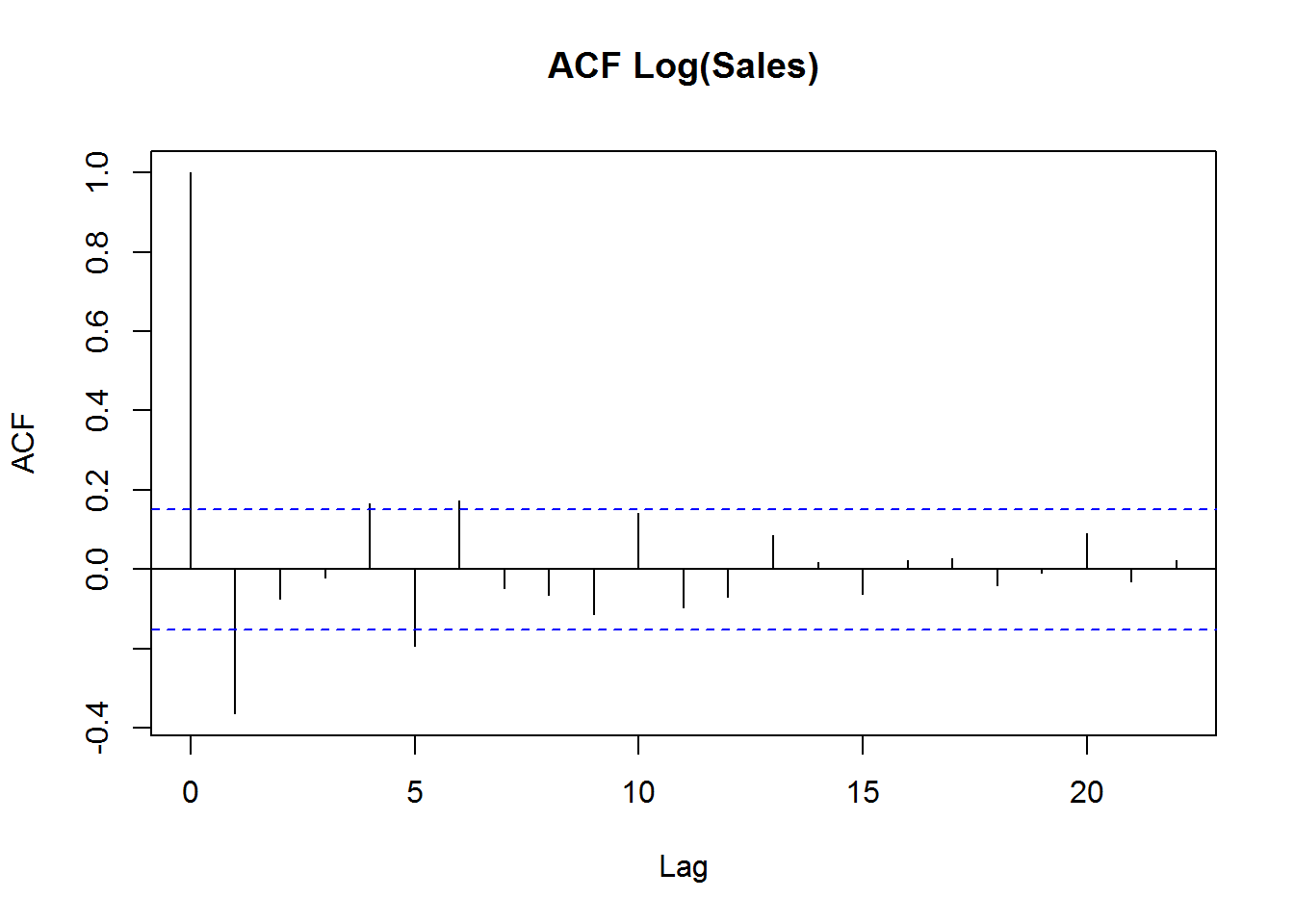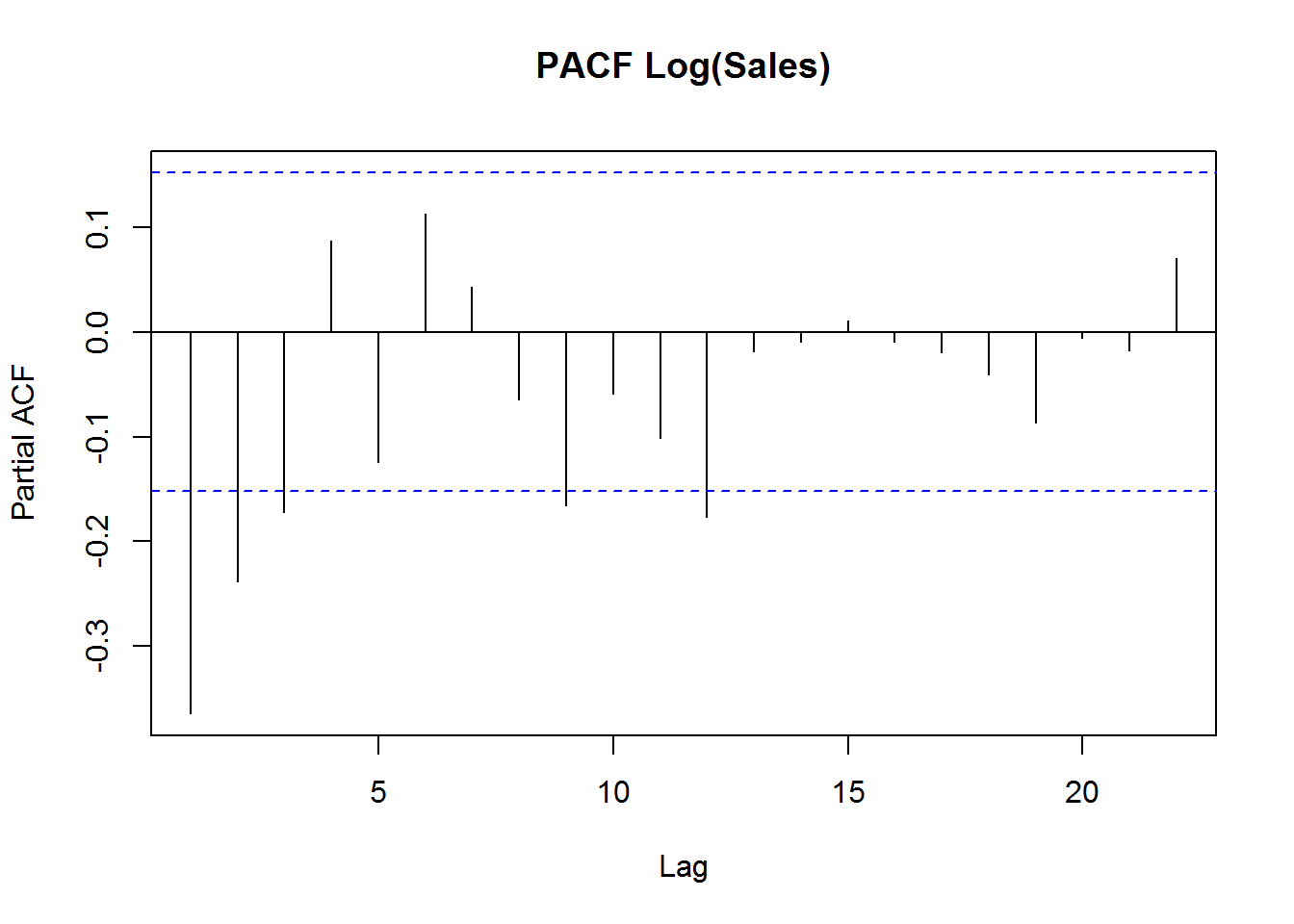Next we auto fit the arima model on 2nd Order Difference of the series “Log(Sales)”. So we effectively use the 1st Order Difference of the series “Log(Sales)” as the variable.

This fitting is given a name of “ARIMAautofit2”. The best model that can be fitted in this case is determined automatically to be ARIMA(0,1,1). ARIMA(0,1,1) model implies this is a purely Moving Average Series.
Note: We have intentionally not performed the manual fitting as it has already been explained in the previous case. Now we need to get a summary of the latest best fit model named as “ARIMAautofit2”.

The summary gives us the coefficients of the best fit model equation. We take a note of the values of the different “Training set error measures”. Here we see that the value of RMSE for this fit is 0.0048 (approx.).

However we cannot compare the 2 best fits represented by ARIMAautofit ~ ARIMA(1,0,1)(0,0,1) and ARIMAautofit2 ~ ARIMA(0,1,1). This is because ARIMAautofit is a perfect Auto Regressive Moving Average process. While ARIMAautofit2 is a purely Moving Average Process.

We need to check which one is a better fit graphically after making predictions using them. Getting the most accurate Predicted Results using a fitted model is always our target. A fitted model which does not predict accurately is useless.

So, we will use both the models viz. “ARIMAautofit” and “ARIMAautofit2” to make separate predictions.
As ARIMAautofit ~ ARIMA(1,0,1)(0,0,1) , it is expected to give us the better predictive results.
But as ARIMAautofit2 ~ ARIMA(0,1,1) i.e. actually an MA(1) process, it is expected that using the Exponential Smoothing Method would increase the predictive power of this model.

### Prediction using ARIMAautofit ~ ARIMA(1,0,1)(0,0,1)

Here we make predictions for the next 36 months, i.e. for a stretch of 3 years in the future. For this,  we use the option “n.ahead = 36”. We store the results of this under the name “pred”. To see the Predicted Values we use the following.

To see the Standard Errors of the Predicted Values we use the following.

Next, we go for plotting the observed and the predicted data together, using “ARIMAautofit”
This will help us to get a visual idea about the accuracy of the fit.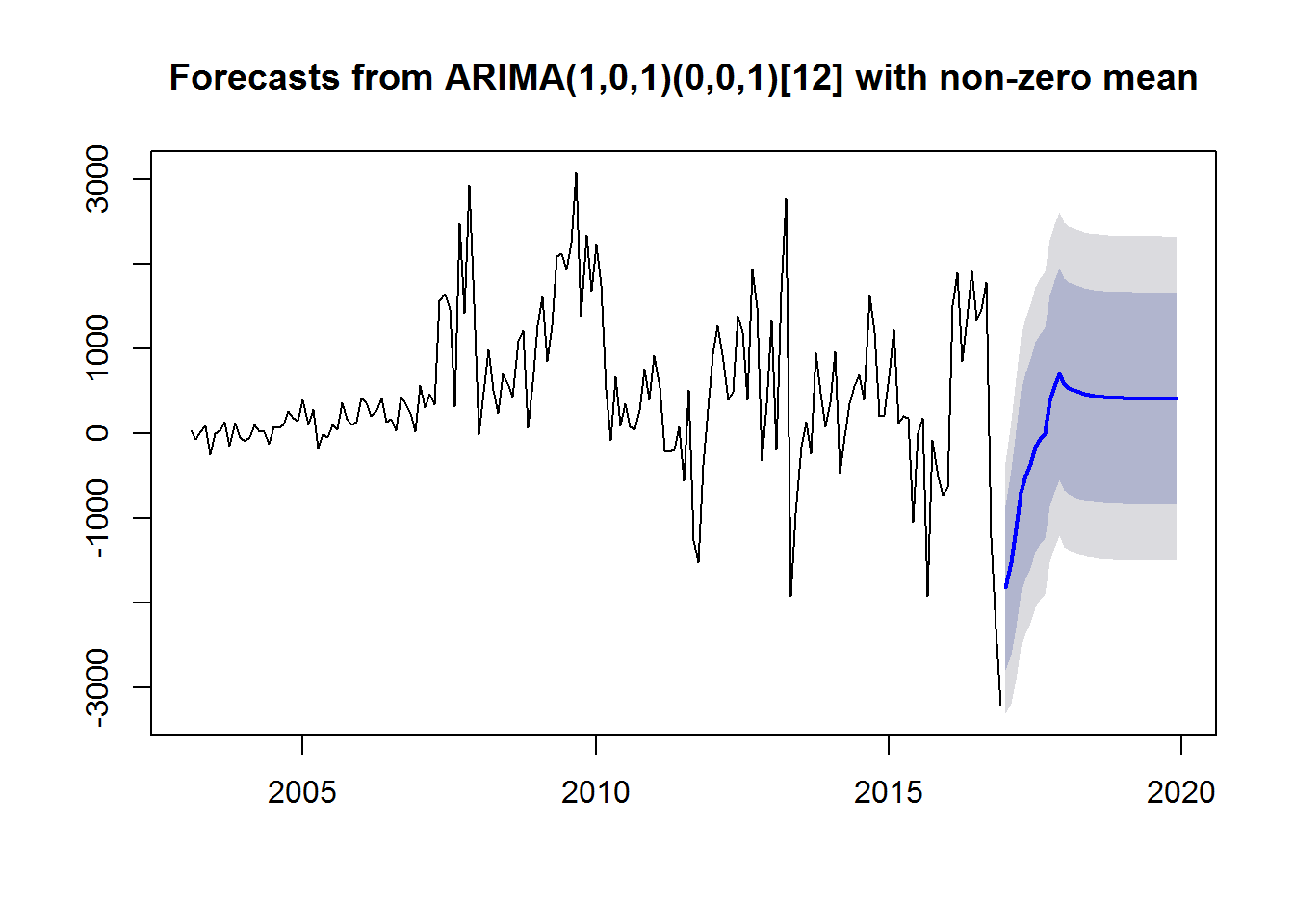We extend the plot by forecasting the values for the next 36 months i.e. the next 3 years. The predictions, in this case, have been done starting from Jan-2017 to Dec-2019. The plot shows the predicted values of the series with a blue line.

The predicted line shows an upward trend starting from Jan-2017 till Dec-2017, after which its falls for a while and then flattens out. The inner purple zone shows the 80 % Confidence Interval of our prediction.
The inner Purple zone added with the outer Grey zone together shows the 95 % Confidence Interval of our prediction.

It can be seen that the Confidence Intervals become wider gradually over the prediction range. They keep on widening from the Jan-2017 till Dec-2017, after which they become constant through out. So, it is apparent that the forecast done here is not that accurate after 1 year.

### Residual Analysis

Next we go for a mandatory Residual Analysis of ARIMAautofit. We look at the ACF and the PACF plots of ARIMAautofit.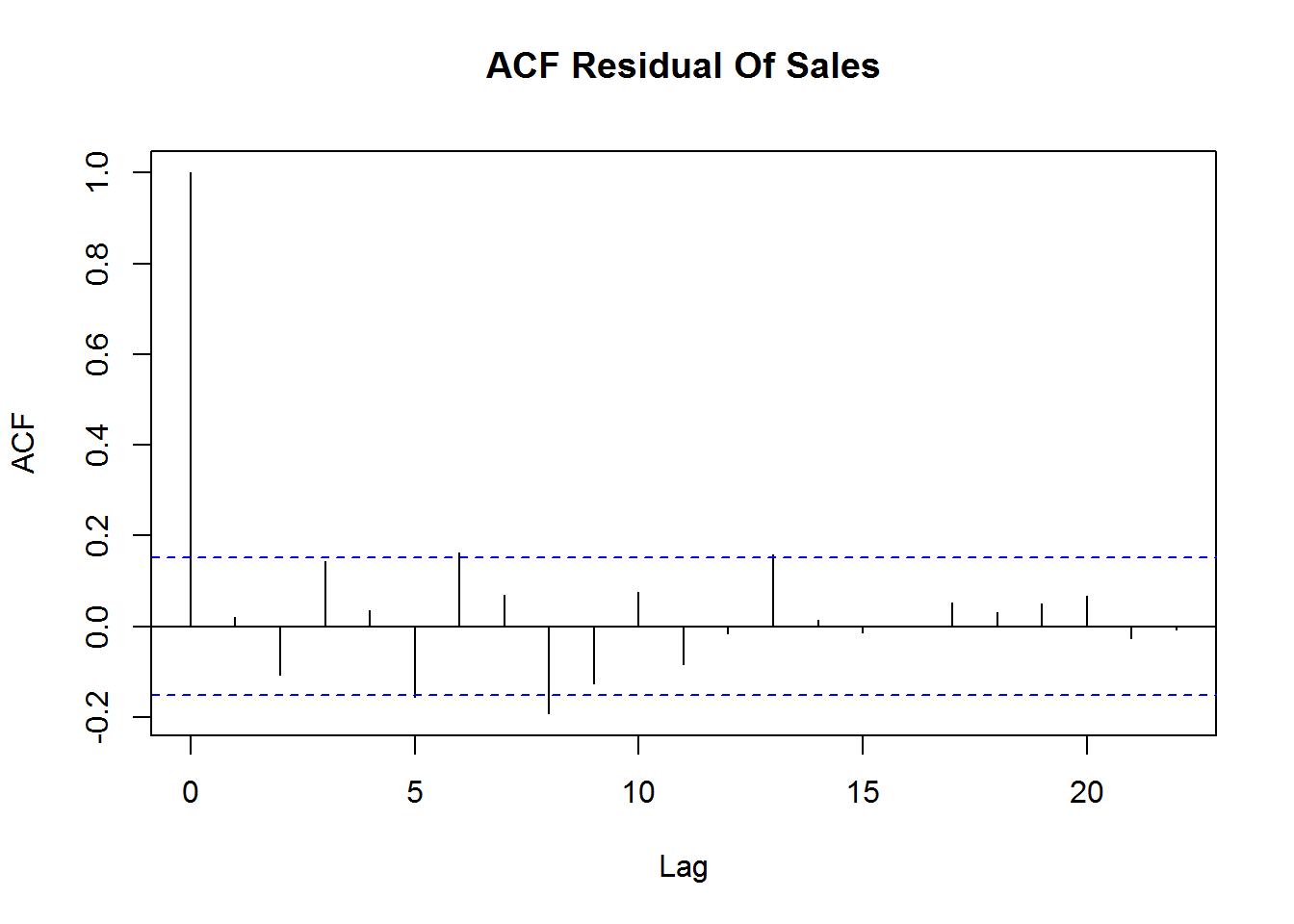The ACF graph shows that the spikes are within the acceptable limits at all lags. So, there is no Moving Average pattern prevalent in the Residuals.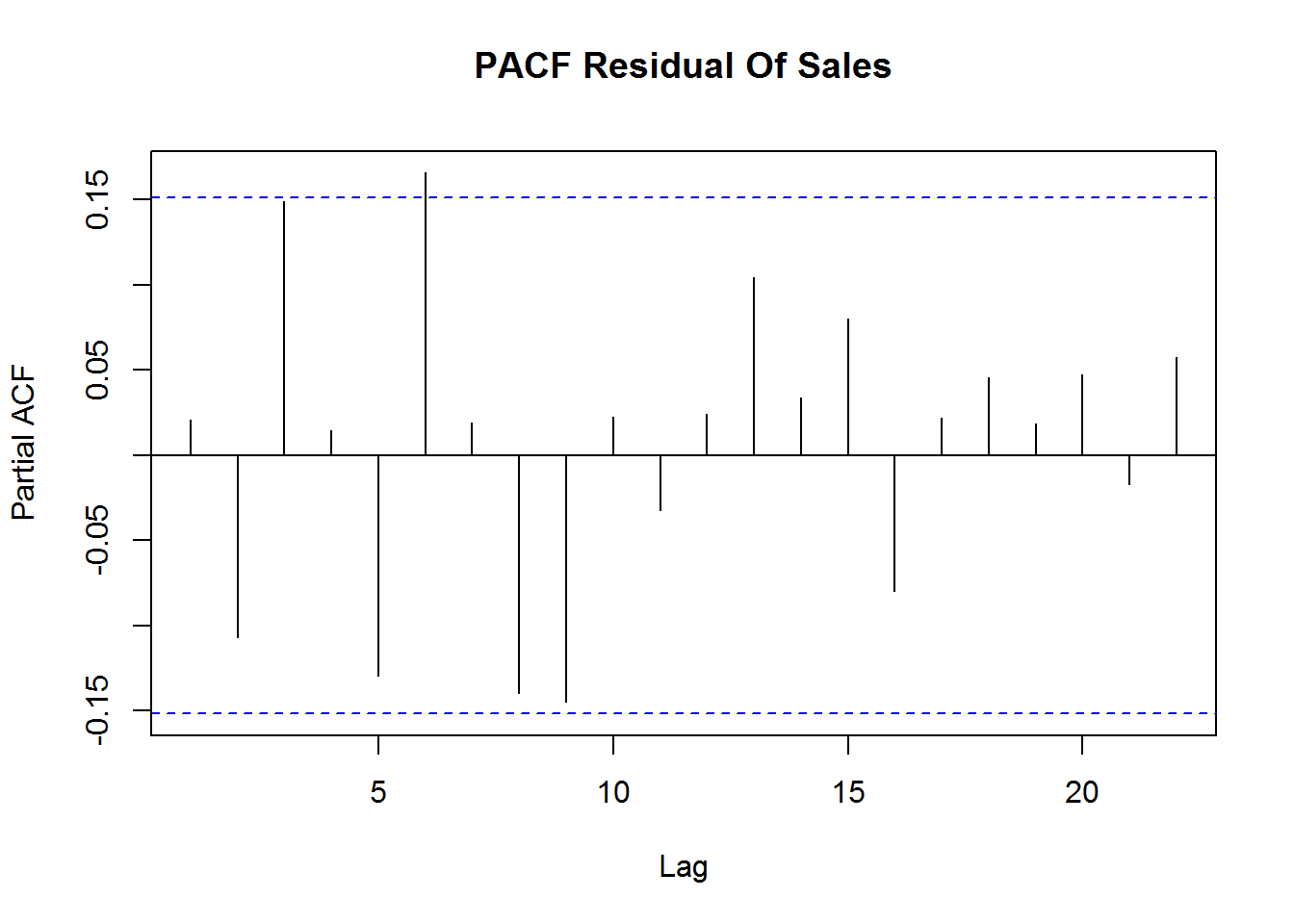The PACF graph shows that the spikes are within the acceptable limits at all lags. So, there is no Auto Regressive pattern prevalent in the Residuals. Thus due to the absence of both AR and MA patterns in the Residuals, we can say that the Residuals, in this case, are random in nature, which is actually desired.

### Prediction using ARIMAautofit2 ~ ARIMA(0,1,1).

Here it is evident that ARIMAautofit2 actually ~ MA(1). Thus it is a pure Moving Average process. Thus instead of fitting it with an ARIMA model, it is desirable to re-fit it into an MA structure.

For this, we will be using Exponential Smoothing. For our convenience and to save time, we refrain from carrying out the step by step process of Simple Exponential Smoothing, Double Exponential Smoothing and Triple Exponential Smoothing. Instead, we go for an Automatic Exponential Smoothing using the State Space Model approach.

Here we perform an Exponential Smoothing by using the “ets( )” function. We store the newly fitted model by the name “autoexp_fit”. Next we go look into the summary of this fit to make some decisions.

From the results, we get an idea about the values of the different “Training set error measures”.
We see that this fit has an RMSE of 0.0047. We note down this RMSE value for “autoexp_fit” and compare it with the RMSE values obtained from several other model fits which also have undergone Exponential Smoothing.

The model fit with the lowest RMSE value, obtained after Exponential Smoothing, will be considered as the best fit. We see that earlier in case of “ARIMAautofit2” which was not fitted after Exponential Smoothing, the RMSE was obtained as to be equal to 0.0048.

But now, under Exponential Smoothing, we find the RMSE value for “autoexp_fit” to be 0.0047. So, there has been no significant increase in the accuracy of the model in this case. We go for predicting using this “autoexp_fit”, for the next 36 months. Thus we would be forecasting the values for the next 3 years, i.e. from Jan-2017 till Dec-2019.

Next we go for plotting the observed and the forecasted data together, using “autoexp_fit”
This will help us to get a visual idea about the accuracy of the fit.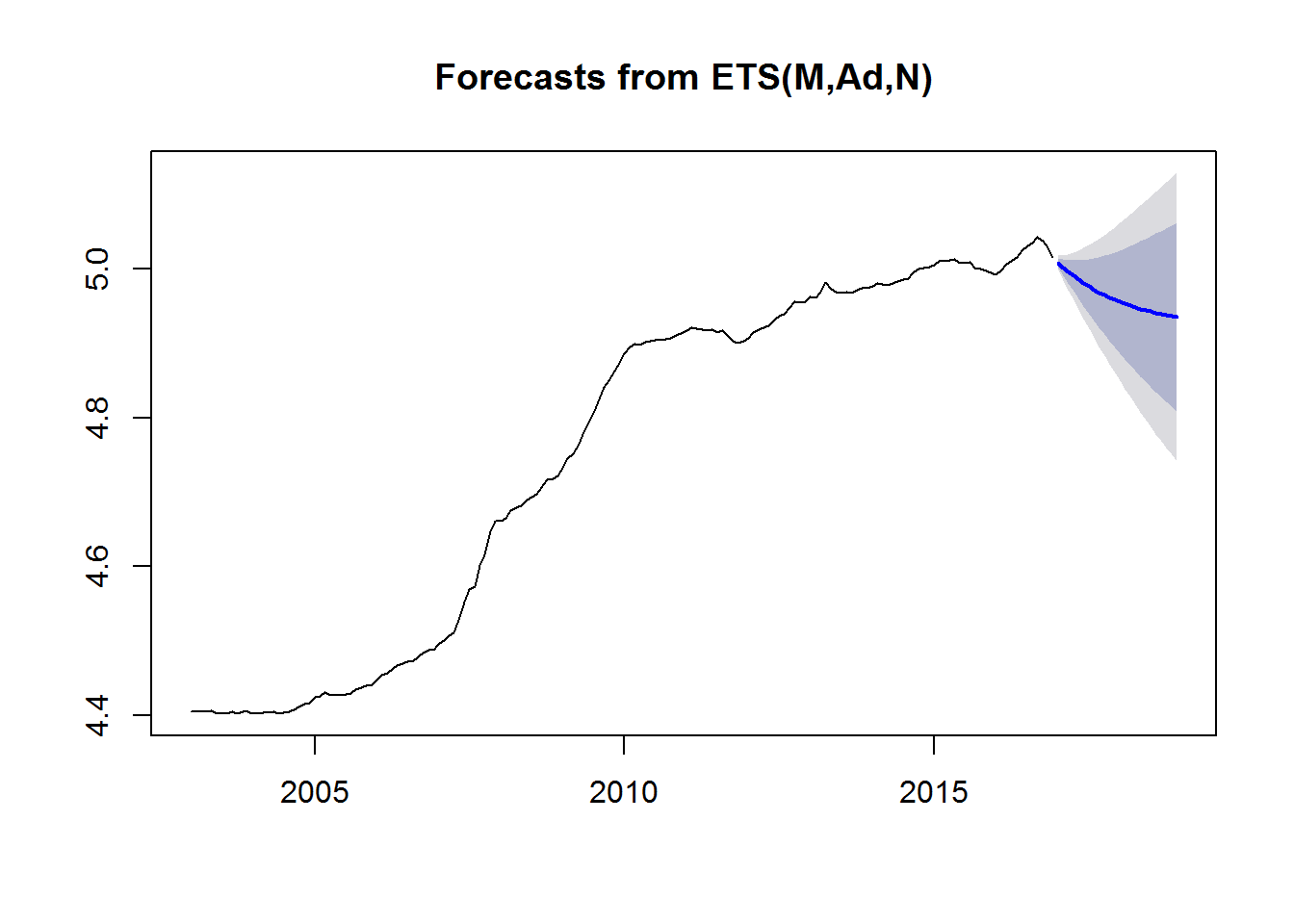From the graph, we see that the predicted value of “Sales”, given by the deep Blue line, declines gradually from Jan-2017 to Dec-2019. The inner purple zone shows the 80 % Confidence Interval of our prediction.
The inner Purple zone added with the outer Grey zone together shows the 95 % Confidence Interval of our prediction.

It can be seen that the Confidence Intervals become wider gradually over the prediction range. So, it is apparent that the accuracy of the forecast declines gradually with time. Next, we go for a mandatory Residual Analysis of “autoexp_fit”. We look at the ACF plot of “autoexp_fit”.

Here as this is a purely MA process, no PACF plot is required.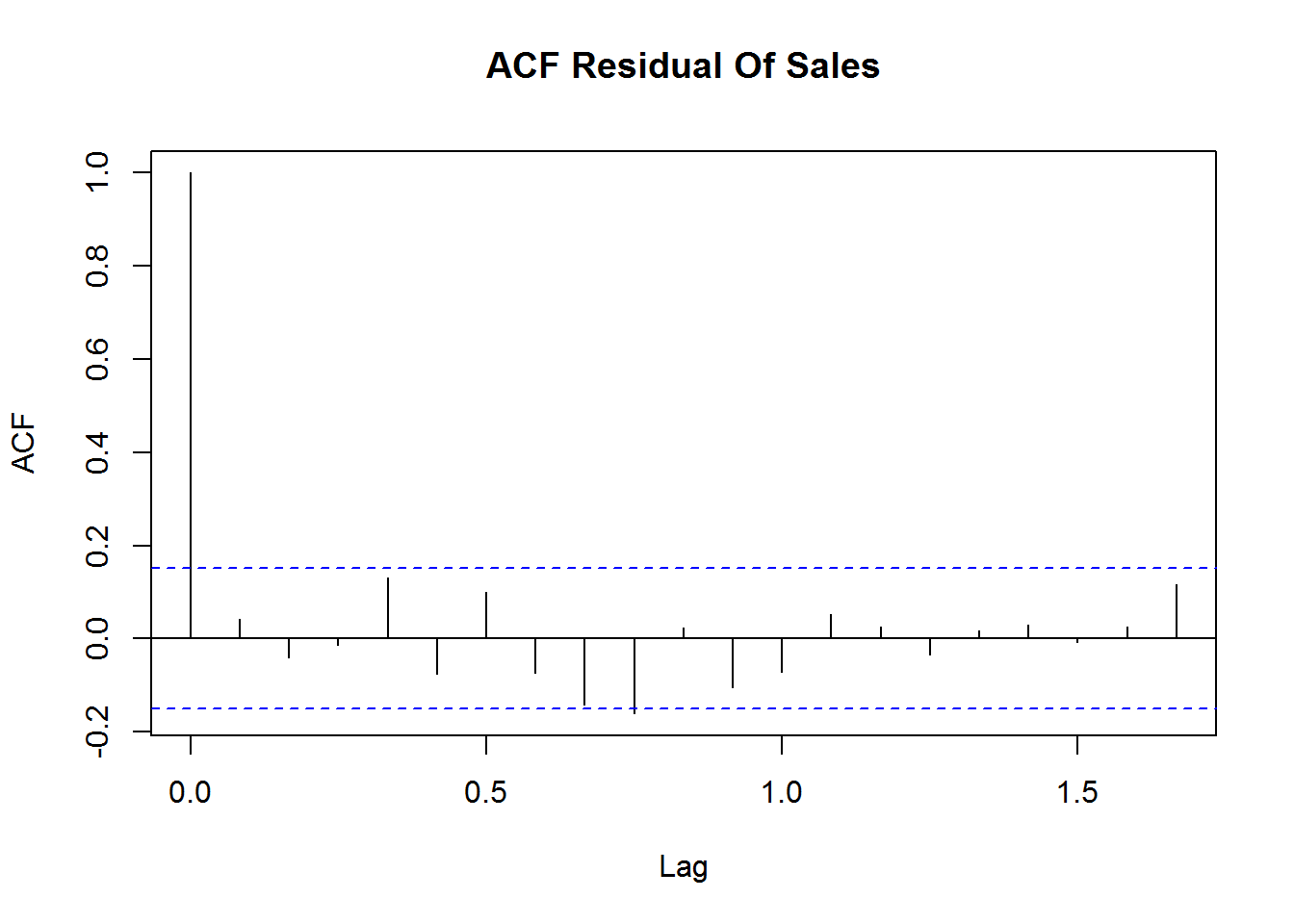The ACF graph shows that the spikes are within the acceptable limits at all lags. So, there is no Moving Average pattern prevalent in the Residuals. It can be said that the Residuals are random in nature, which is actually desired.

Thus we show how an analysis of Time Series Data can be done accurately. At last, just to recapitulate we again recall that a proper ARIMA series is better fitted using the “auto.arima( )” process, while in most cases a purely MA series is better fitted using the Exponential Smoothing Method of “ets( )”.